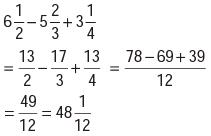Courses

# Olympiad Test : Fractions - 1

## 10 Questions MCQ Test Maths Olympiad Class 6 | Olympiad Test : Fractions - 1

Description
This mock test of Olympiad Test : Fractions - 1 for Class 6 helps you for every Class 6 entrance exam. This contains 10 Multiple Choice Questions for Class 6 Olympiad Test : Fractions - 1 (mcq) to study with solutions a complete question bank. The solved questions answers in this Olympiad Test : Fractions - 1 quiz give you a good mix of easy questions and tough questions. Class 6 students definitely take this Olympiad Test : Fractions - 1 exercise for a better result in the exam. You can find other Olympiad Test : Fractions - 1 extra questions, long questions & short questions for Class 6 on EduRev as well by searching above.
QUESTION: 1

### The smallest of the fractions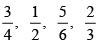is

Solution:

Here, LCM of 4, 2, 6, 3 =12.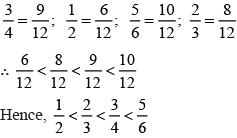QUESTION: 2

### The largest of the fraction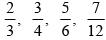is

Solution:

LCM of 3, 4, 6, 12 = 12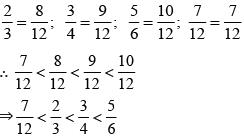QUESTION: 3

### If 5/6 is equivalent to X/24, then what is the value of X?

Solution: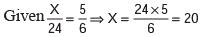QUESTION: 4

Which of the following is a proper fraction?

Solution:
QUESTION: 5

A fraction equivalent to 57/95 is

Solution: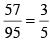QUESTION: 6

A fraction equivalent to 2/3 is

Solution:
QUESTION: 7

What should be added to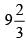to get 19?

Solution:

Required value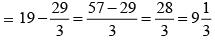QUESTION: 8

Which of the following is not a proper fraction?

Solution:
QUESTION: 9

Raju bought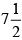litres of milk. Out of this milk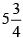litres was consumed. How much milk is left with him?

Solution:

Required amount of milk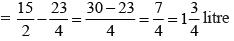QUESTION: 10

What is the value of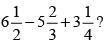Solution: